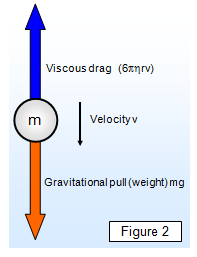# Stokes' law and terminal velocityWhen any object rises or falls through a fluid it will experience a viscous drag, whether it is a parachutist or spacecraft falling through air, a stone falling through water or a bubble rising through fizzy lemonade. The mathematics of the viscous drag on irregular shapes is difficult; we will consider here only the case of a falling sphere. The formula was first suggested by Stokes and is therefore known as Stokes' law.

Consider a sphere falling through a viscous fluid. As the sphere falls so its velocity increases until it reaches a velocity known as the terminal velocity. At this velocity the frictional drag due to viscous forces is just balanced by the gravitational force and the velocity is constant (shown by Figure 2).

At this speed:

Viscous drag = 6πηrv = Weight = mg

The following formula can be proved (see dimensional proof)Frictional force (F) = 6πηrv    (Stokes' law)

If the density of the material of the sphere is r and that of the liquid σ, then:

Effective gravitational force = weight - upthrust = 4/3πr3(ρ – σ)g]

Therefore we have for the viscosity (η):

Viscosity (η) = 2gr2(ρ - σ)/9v

Stokes’ law shows that the frictional drag (F) is directly proportional to the weight of the sphere; in other words F is proportional to r3. The formula for viscosity shows that the terminal velocity (v) is proportional to the radius squared; v is greater for a larger sphere than for a smaller one of the same material.

Stokes' law is important in Millikan's experiment for the measurement of the charge on an electron, and it also explains why large raindrops hurt much more than small ones when they fall on you - it's not just that they are heavier, they are actually falling faster.

People falling through the atmosphere will experience frictional drag due the air, eventually reaching their terminal velocity. For low-level air (below about 3000 m) this is around 200 km/hour flat out and just over 320 km/hour head down. However at high altitudes around 30 000m this can reach almost 1000 km/hour!

Figure 3 shows how the velocity of an object will increase with time as it falls through a viscous fluid.

It is interesting to consider the effect of various shapes of objects falling through a fluid. These can be made from plasticene.

See the section on subsonic, supersonic and hypersonic vehicles and the shape of the bulbous bow on a nuclear powered submarine.### schoolphysics Stokes' Law animation

To see an animation of Stokes' Law click on the animation link.

A VERSION IN WORD IS AVAILABLE ON THE SCHOOLPHYSICS USB